Metonymy and Metaphor in Mathematics

## 5. Metaphors from modern mathematics: II. The Jordan normal form and the structure of abelian groups

Twomatrices A and B are similar if there is an invertiblematrix S such that B=S-1AS. This means that A and B are doing the same thing'' but seen with respect to two different sets of basis vectors.

Anymatrix A with complex entries is similar to a matrix in Jordan normal form. This is a matrix of the form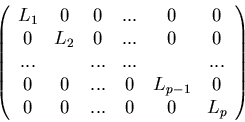where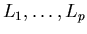are square blocks of various sizes adding up to n, arranged along the diagonal, and the 0s represent appropriate blocks of zeroes. Furthermore each Li has the form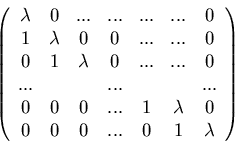for some complex number.

The metaphier. The structure theorem for finite abelian groups says that a finite abelian G is isomorphic to a direct sum of cyclic groups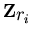, where ri is a power of a prime, and that this decomposition is unique up to ordering. For example, an abelian group of order 12 must be isomorphic to either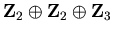or. There are no other possibilities. This generalizes to a structure theorem for modules over a principal ideal domain.and the ring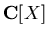of complex-valued polynomials are both p.i.d.s; an abelian group is naturally a-module, with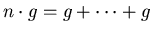(n times).

The metaphor. Use A to make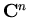into a-module by letting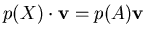so, etc. The analogue ofmod the multiples of ri (a prime power) ismod the multiples of, since inthe irreducible polynomials are linear.

By the structure theorem,splits up into subspaces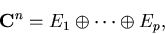and restricted to Ei the module structure is the same as the action ofon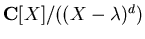, for some complex. A basis for the quotient isWith respect to this basis the matrix of A, which corresponds to multiplication by X, comes out as follows:giving exactly the block Li described above.

(For more details see a text like Hartley and Hawkes, Rings, Modules and Linear Algebra,Chapman & Hall, London, New York, 1970 or Serge Lang, Algebra, Addison-Wesley 1971.)